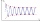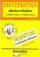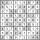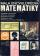# Last page

Two consecutive sheets dropped out of the book. The sum of the numbers on the sides of the dropped sheets is 154. What is the number of the last page of the dropped sheets?

x =  40

### Step-by-step explanation:

x+(x-1)+(x-2)+(x-3) = 154

4x = 160

x = 40

Our simple equation calculator calculates it.Did you find an error or inaccuracy? Feel free to write us. Thank you!Tips to related online calculators
Do you have a linear equation or system of equations and looking for its solution? Or do you have a quadratic equation?

## Related math problems and questions:

• Dropped sheetsThree consecutive sheets dropped from the book. The sum of the numbers on the pages of the dropped sheets is 273. What number has the last page of the dropped sheets?
• A bookA book contains 524 pages. If it is known that a person will select any one page between the pages numbered 125 and 384, find the probability of choosing the page numbered 252 or 253.
• Sum of the seventeen numbersThe sum of the 17 different natural numbers is 154. Determine the sum of the two largest ones.
• Sum of four numbersThe sum of four consecutive natural numbers is 114. Find them.
• BookCalculate the thickness of the page of the collection of examples from mathematics, where 4.87 cm thick and has 451 pages.
• Unknown numberFind the unknown number equal to a quarter of a fifth of a number, which is by 152 more than an unknown number.
• Even / odd numbersa / Using variable n write two consecutive odd numbers. b / the sum of three consecutive odd numbers is 333. What are this numbers?
• 1 pageOne page is torn from the book. The sum of the page numbers of all the remaining pages is 15,000. What numbers did the pages have on the page that was torn from the book?
• There 14There are 250 people in a museum. 2/5 of the 250 people are girls 3/10 of the 250 people are boys The rest of the 250 people are adults Work out the number of adults in the museum.
• The sumThe sum of five consecutive odd numbers is 75. Find out the sum of the second and fourth of them.
• BookTo number pages of the thick book was used 4201 digits. How many pages has this book?
• RemaindersIt is given a set of numbers { 170; 244; 299; 333; 351; 391; 423; 644 }. Divide this numbers by number 66 and determine set of remainders. As result write sum of this remainders.
• Sum of sevenThe sum of seven consecutive odd natural numbers is 119. Determine the smallest of them.
• Have solutionThe sum of four consecutive even numbers is 92. Determine these numbers.
• How manyHow many numbers are less than 222 with a digit sum is 8?
• Unknown numbersThe sum of two consecutive natural numbers and their triple is 92. Find these numbers.
• Page numberingThe book has 88 pages. How many times is the number 4 used for the book numbering?